# 4-3 Measurement of Interface Efficiency

 Team-Fly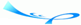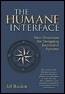Human Interface, The: New Directions for Designing Interactive SystemsBy Jef Raskin Table of Contents Chapter Four.  Quantification

Every tool carries with it the spirit by which it has been created.

?span>Werner Karl Heisenberg

We have looked at two interfaces, one of which will take about 5 seconds to operate and the other of which will take more than 15 seconds to operate. It is clear which of the two better satisfies the requirement. The next question that we ask is how fast an interface that satisfies the requirement can be.

Given a design for an interface, you can use GOMS and its extensions to calculate how long a user will take to accomplish any well-defined task with that interface. But analysis models do not answer the question of just how fast you should expect an interface to be. To answer this question, we can use a measure from information theory. In the following discussion, information is used in the technical sense of a quantification of the amount of data conveyed by a communication, such as when two people have a telephone conversation or when a human sends a message, such as a click of the GID button when the cursor is at a certain location, to a machine. Before dealing with the technical details of measuring the amount of information a user must provide to accomplish a task, we establish the need for such a measurement.

To make a reasonable estimate of the time that the fastest possible interface for a task would take, we can proceed by first determining a lower bound on the amount of information a user has to provide to complete that task; this minimal amount is independent of the design of the interface. If the methods of a proposed interface require an input of information that exceeds the calculated lower bound, the user is doing unnecessary work, and the proposed interface can be improved. On the other hand, if the proposed interface requires the user to supply exactly the amount of information that the task requires, you cannot make a more information-efficient interface for this task. In this latter case, there may yet be ways of improving and there are certainly many ways of ruining the interface, but at least this one efficiency goal will have been met.

Information-theoretic efficiency is defined similarly to the way efficiency is defined in thermodynamics; in thermodynamics we calculate efficiency by dividing the power coming out of a process by the power going into that process. If, during a certain time interval, an electrical generator is producing 820 watts while it is driven by an engine that has an output of 1,000 watts, it has an efficiency of 820 / 1,000, or 0.82. Efficiency is also often expressed as a percentage; in this case, the generator has an efficiency of 82 percent. A perfect generator which by the second law of thermodynamics cannot exist would have an efficiency of 100 percent.

The information efficiency E of an interface is defined as the minimum amount of information necessary to do a task, divided by the amount of information that has to be supplied by the user. As is true of physical efficiency, E is at least 0 and is at most 1. Where no work is required for a task and no work is done, the efficiency is defined as 1. (This formality is necessary to avoid the case of 0 divided by 0, as in responding to a transparent error message. See Section 5-5.)

E can be 0 when the user is required to provide information that is totally unnecessary (Figure 4.4). Surprisingly, a number of interface details achieve the dubious honor of having E = 0. A dialog box that allows the user only one possible action, such as clicking the box's OK button, is such an example. (JavaScript has a command, Alert, solely for creating such unnecessary boxes: The designers were wise enough to remove goto from the JavaScript language to force structured code, but they failed to provide similar guidance on the interface side.)

##### Figure 4.4. A dialog box with an information theoretic efficiency of 0.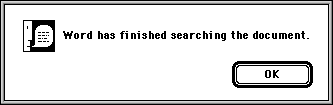E takes into account only the information required by the task and that supplied by the user. Two or more methods may have the same E, yet have different total times. It is even possible that a first method has a higher E yet is slower than a second method for example, M K M K versus M K K K. In this example, only two characters have to be entered when the first method is used. In the second method, three characters are required, yet it takes less time to perform the task. It is difficult to construct many real-life situations that exhibit this inversion of speed and information efficiency. For the most part, the more efficient interface is also the more productive, more humane interface.

 It is possible to design more sophisticated measures of efficiency; for example, the M operator does not enter into our calculation. However, the simple measure defined here suffices for the purposes of this book.

Information is measured in bits; a single bit, which represents a choice between two alternatives such as 0 and 1, on and off, or yes and no is the unit of information. For example, a choice made among four objects would require 2 bits of information: If the objects are A, B, C, and D, the first bit could choose either A and B, or C and D; once that choice was made say C and D the second bit would choose either C or D. Two binary choices, or 2 bits, suffice to separate one item from a set of four. To choose among eight alternatives, you need 3 bits; to choose among sixteen items, you need 4 bits; and so on. In general, given n equally likely alternatives, the amount of information communicated by all of them taken together is the power to which 2 has to be raised to obtain n:

 Bit is mathematician John W. Tukey's contraction of the words BInary digiT (Shannon and Weaver 1963, p. 9).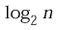And the amount of information in any one of them is

Equation 1If the probabilities among the alternatives are not necessarily equal and the ith alternative has probability p(i), the information associated with that alternative is

Equation 2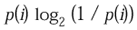The amount of information is the sum (over all alternatives) of expression (2), which reduces to expression (1) in the equiprobable case. It follows that the information content of an interface that allows only the tap of a single button is 0 bits; not tapping the button is not permitted:

Equation 3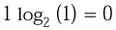It would seem, however, that the required tap of a single button can, for example, cause the ignition of dynamite used to demolish a building. Would this tap of the button then convey information? It would not, because not tapping the button was not an alternative; the interface "allows only the tap of a single button." If, however, the button was not tapped during, say, a five-minute time window in which the demolition was permitted, the building would not be demolished, and the tap or nontap would convey up to 1 bit of information because there were, in this case, two possible messages. From expression (2), we know that the calculation involves the probability, p, that the building will be exploded. The probability that it will not be exploded is therefore 1 ?p. From expression (2), we can calculate the information content of this interface:

Equation 4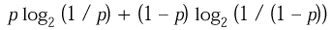When p = ½, expression 4 evaluates to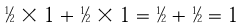Expression (4) evaluates to less than 1 if p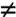½. In particular, it evaluates to 0 when p = 1, as in expression (3).

This example illustrates an important point: We can measure the information embodied in a message only in the context of the set of possible messages that might have been received. To calculate the amount of information that has been conveyed by the reception of a message, we must know, in particular, the probability of that message having been sent. The amount of information in any message is independent of other messages past or future, is without reference to time or duration, and does not depend on any other events; similarly, the outcome of the flip of a fair coin is unaffected by previous tosses or by what time of day it is tossed.

As explained in Shannon and Weaver (1963), it is also important to keep in mind that

information should not be confused with meaning...information is a measure of one's freedom of choice when one selects a message....Note that it is misleading (although often convenient) to say that one or the other message [when just two are possible] conveys [1 bit of] information. The concept of information applies not to the individual messages (as the concept of meaning would), but rather to the situation as a whole, the unit information indicating that in this situation one has an amount of freedom of choice in selecting a message, which it is convenient to regard as a standard or unit amount. (p. 9)

However, a user's actions in performing a task could be modeled with greater accuracy as a Markoff process, whereby the probability of a later action depends on earlier actions taken by the user, but the single-event probabilities discussed are sufficient for the purposes of this book; messages are assumed to be independent and equiprobable.

The amount of information conveyed by nonkeyboard devices can also be calculated. If your display is divided into two regions one labeled Yes and the other labeled No a single click in one or the other region would supply 1 bit of information. If there are n equally likely targets, with one click, you supply log2 n bits of information. If the targets are of unequal size, the amount of information given by each does not change, but it does take longer to move the GID to smaller targets by an amount that we shall show how to calculate presently. If the targets have unequal probability, the formula is the same as that already given for keyboard inputs with unequal probabilities. There is a difference in that a user can operate a keyboard key in 0.2 sec, whereas it will take 1.3 sec to operate an on-screen button, on average, ignoring homing time.

For our purposes, we can calculate the information content of voice input by treating speech as a sequence of input symbols, rather than as a continuous phenomenon with a certain bandwidth and duration.

This treatment of information theory and its relationship to interface design is a simplified account. Yet even in this rudimentary form, information theory used in a manner analogous to our use of the simplified GOMS keystroke-level model can give us first-order guidance in evaluating the quality of our interface designs.

#### 4-3-1 Efficiency of Hal's Interfaces

Accurate reckoning: The entrance into the knowledge of all existing things and obscure secrets.

?span>Rhind Papyrus, c. 1650 B.C.

It is useful to go through a detailed example of a calculation of the average amount of information required for an interface technique. I will again use the temperature-conversion example. According to the requirement, the input needed by the converter consists of an average of four typed characters; a decimal point occurs once in 90 percent of the inputs and not at all in the other 10 percent, and the negative sign occurs once in 25 percent of the inputs and not at all in the other 75 percent. For simplicity, and because there is no need for 1 percent precision in the answer, I will assume that all of the other digits occur with equal frequency, and I will ignore the 10 percent of the inputs that have no decimal point.

We need to determine the set of possible messages and the probability of each. Five forms are possible, where d denotes a digit:

1. ?dd

2. ?span>d.d

3. .ddd

4. d.dd and

5. dd.d.

The first two each occur 12.5 percent of the time, and there are 100 of each of them; the final three each occur 25 percent of the time, and there are nearly 1,000 of each. The probability for either of the first two types of messages is (0.125 / 100) = 0.00125; the probability for any one of the final three types of messages is (0.75 / 3000) = 0.00025. The sum of the probabilities of the messages is, as it must be, 1.

 The "nearly" comes from the fact that the temperature of 0 degrees will not be entered as 0.00 or 00.0.

The amount of information of each message, in bits, is given by expression (2):

 To get logs to the base 2 on a calculator or a computer that has only natural logs (ln), use: log2 (x) = ln (x) / ln (2).

p(i) log2 (1 / p(i))

This expression evaluates to approximately 0.012 for the negative values and to 0.003 for the positive values. Calculating 200 x 0.012 + 3000 x 0.003 gives a total of 11.4 bits for each message.

Taking the probabilities into account can be important. If we took a simple-minded approach and assumed that all of the 12 symbols (minus, decimal point, and the 10 digits) were equally likely, the probability of each would be 1/12, and the information contained in a four-character message would be approximately

4 log2 (12)14 bits

It is a theorem of information theory that the information is at a maximum when all symbols are equally likely. Therefore, making the assumption of equiprobable messages will give you a value that is equal to or greater than the amount of information in each message. Obviously, this assumption also makes estimating the information content of a message easier to compute. If the resultant value of the approximation is smaller than the amount of information your interface requires the user to supply, you do not yet need to bother with the more refined calculation.

We have just calculated that the task requires that Hal supply an average of about 11 bits of information each time he has to convert a temperature. We can and will, presently divide this quantity by the amount of information the interface requires him to supply. The result will be the efficiency of the interface.

Another simplification for quick analysis is to find the amount of information in a keystroke or a GID operation and then to count the various gestures. When a keystroke delivers information to a computer, the amount of information delivered depends on the total number of keys available for example, the number of keys on the keyboard and the relative frequency with which each key is used. Thus, keystrokes can be used as a rough measure of information. If a keyboard had 128 keys, each of which had the same frequency of use, each key would represent 7 bits of information. In practice, the frequency of use varies tremendously for example, space and e are common, whereas j and \ are rare), and the information per keystroke is closer to 5 bits in most applications. The requirement stated that the average length of the input that specifies the temperature was four keystrokes.

For this analysis, it is easier to use a measure simpler than information-theoretic efficiency but that often achieves the same practical effect. Character efficiency is defined as the minimum number of characters required for a task, divided by the number of characters the interface makes the user enter.

Achieving an interface that required four keystrokes, on average, would give us a character efficiency of 100 percent. If we add a keystroke to decide which conversion is desired and then another to delimit the answer, our average length of input will grow to six keystrokes, and our keystroke efficiency will drop to 67 percent. If Hal has as his input device only a 16-key numeric keypad, the information provided by a single keystroke would be 4 bits, and the interface would be more efficient. (The requirements, however, do not permit us to use this option.)

Because any task in a GOMS analysis requires at least one mental operator, the most keystroke-efficient interface for the temperature-conversion problem will have, in theory, an average time of

M + K + K + K + K = 2.15 sec

Thus, it will be considerably faster than either of the two interfaces already discussed. However, typing four characters on a standard keyboard supplies at least 20 bits of information, whereas only 11 bits are required an information-theoretic efficiency of 55 percent so we know that there is room for improvement. As we have seen, using a standard numeric keypad instead of a full keyboard drops the input information per four keystrokes to 16 bits, raising the efficiency to about 60 percent. A dedicated numeric keypad one that has only the digits, the minus sign, and a decimal point will permit a slightly higher score, of about 70 percent efficiency. We raise the score again by using special encodings of temperature information and novel input devices, but training difficulties and excessive costs begin to loom with these extreme approaches, so I will stop here and accept 70 percent information-theoretic efficiency. Theoretical limits may or may not be reached by a practical interface, but they do give us a star by which to steer.

#### 4-3-2 Other Solutions for Hal's Interface

In Section 4-3-1, we stopped trying to improve information-theoretic efficiency when we reached 70 percent. We achieved that efficiency with an unspecified, theoretical interface that somehow managed to have 100 percent keystroke efficiency. Let us see how close we can come to this ideal with a standard keyboard and a GID.

Consider an all-keyboard interface. In this interface, a note appears on the display:

 ` To convert temperatures, indicate the desired scale by typing C for Celsius or F for Fahrenheit. Type the numeric temperature; then press the Enter key. The converted temperature value will be displayed. `

A GOMS analysis finds that the user must make six keystrokes. Following the rules for placements of Ms gives us

M K K K K K M K

The average time is 3.9 seconds.

We can decrease this time if we can use the C or the F itself as a delimiter. That is, consider an interface in which the following instructions appear:

 ` To convert temperatures, type the numeric temperature, followed by C if it is in degrees Celsius or F if it is in degrees Fahrenheit. The converted temperature will be displayed. `

In this example, the Enter key is not used. Some primitive interface-building tools demand that the user tap Enter and will not permit us to use C or F as a delimiter; such tools are inadequate for building humane interfaces.

The GOMS analysis of the C/F-delimiter interface yields

M K K K K M K

The average time is 3.7 seconds. If we did not have an analysis that showed that the theoretical minimum time is 2.15 sec, this solution might strike us as satisfactory. It is considerably more efficient than the ones that we discussed previously, so we might stop here. Tempted by that theoretical minimum, however, we ask whether there is an even faster approach. Consider the interface depicted in Figure 4.5; we might describe it as bifurcated: One input will give us two outputs.

##### Figure 4.5. An interface that does not require a delimiter. A more efficient interface is made possible by taking advantage of character-at-a-time interaction, and by performing both conversions at once.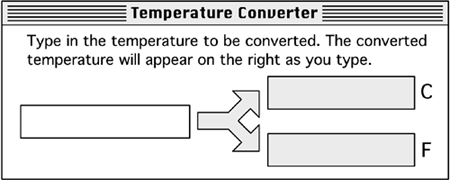Under the bifurcated interface, no delimiter is required. Furthermore, the user does not have to specify which conversion is desired. The GOMS analysis for the average input of four characters is

M K K K K

The bifurcated interface achieves the minimum 2.15 seconds and has 100 percent character efficiency.

If, as in our example, the output sometimes changes when a character is typed, the flickering of the output does not distract you, because your locus of attention is the input. The continually changing output is often beneficial: The user will notice it only peripherally after the first few times that he uses the feature, at which point it will provide him feedback that the system is responding to his input. For single-character interaction to be effective, the system must respond quickly; in particular, the interaction must keep up with the user's typing speed. Only a slow network connection should exhibit this problem.

Although not part of the requirement, you might ask how this converter is "cleared" for the next operation. Does the clear operation add a keystroke? Not necessarily. For example, we could design the interface such that, whenever the operator returns to his background task or goes on to another task, the values in the converter are automatically grayed and the converter becomes inactive. The values shown are not cleared at this time, so that they can be referred to again if necessary. The next input to the converter does clear the old values.

Just because it has optimal speed of operation and is highly efficient, the bifurcated converter is not necessarily the best interface of those discussed or of those possible. Parameters other than speed also are of importance, such as error rate, user learning time, and long-term user retention of the way to use the interface. We should be especially concerned about the error rate of the bifurcated converter, due to Hal's possibly reading the wrong output box, especially because he may have just heard, for example, the word Celsius and thus be required to read out the Fahrenheit line. Nonetheless, the bifurcated converter would definitely be on the short list of interfaces to be tested for the temperature-converter application, and a few others that we have seen solutions that might otherwise have seemed worth a try had we not learned how to do a GOMS analysis would not make the cut.

Whether we use it in a simple keystroke-timing analysis or in a detailed information-theoretic extravaganza, a quantification of the theoretical minimum-time, minimum-character, or minimum-information interface can be a useful guide for our designs. Without a quantitative guide, we are only guessing at how well we are doing and at how much room there is for improvement.

 Team-FlyTopThe Humane Interface. New Directions for Designing Interactive Systems ACM Press Series
ISBN: 1591403723
EAN: N/A
Year: 2000
Pages: 54